Finding equation of tangent/normal when point and curve is given

Chapter 6 Class 12 Application of Derivatives
Concept wise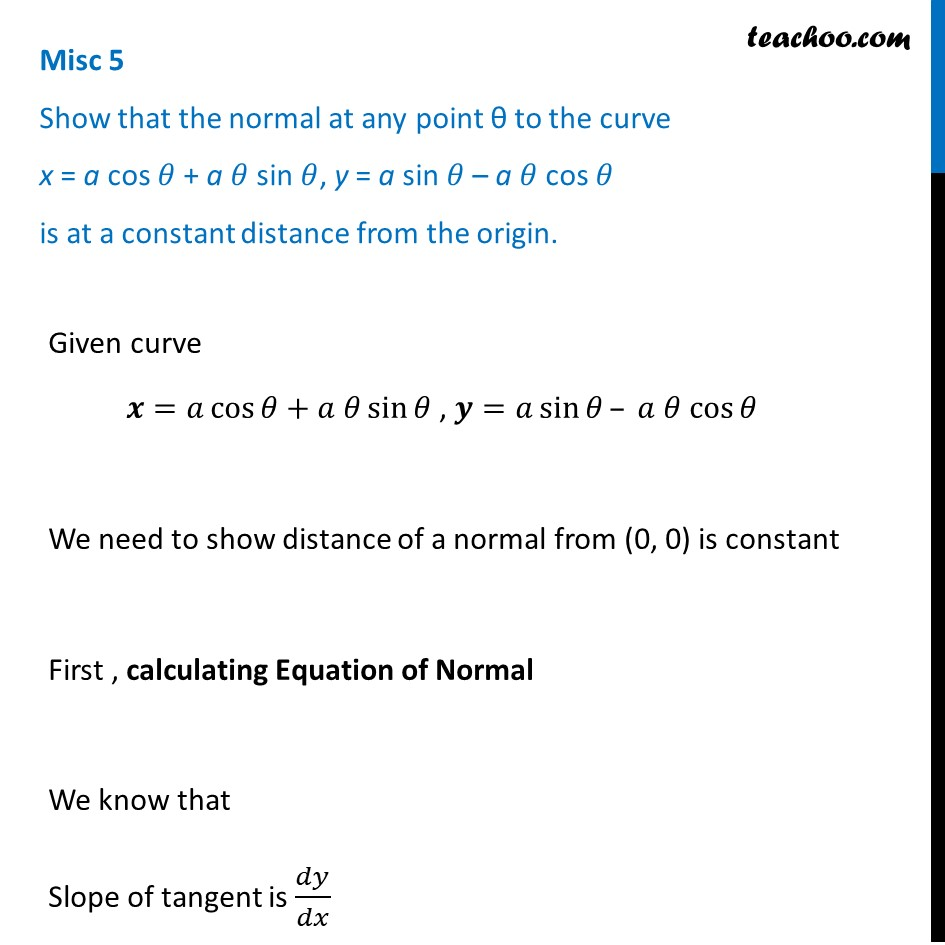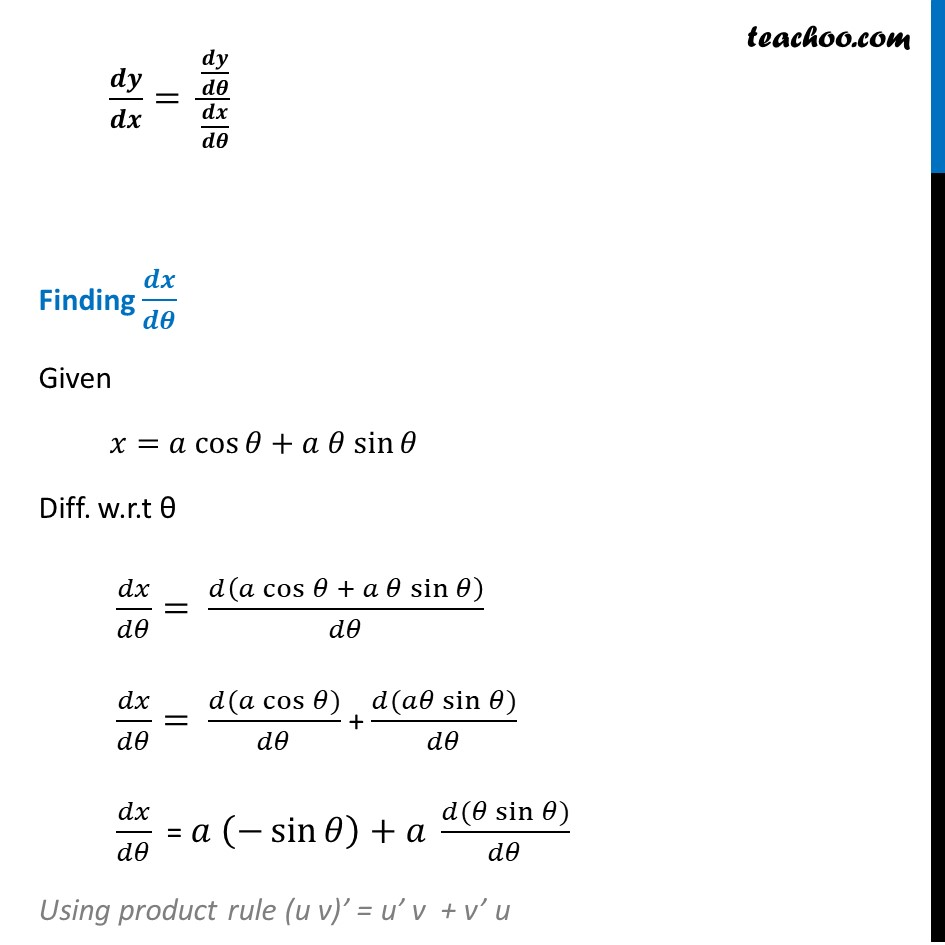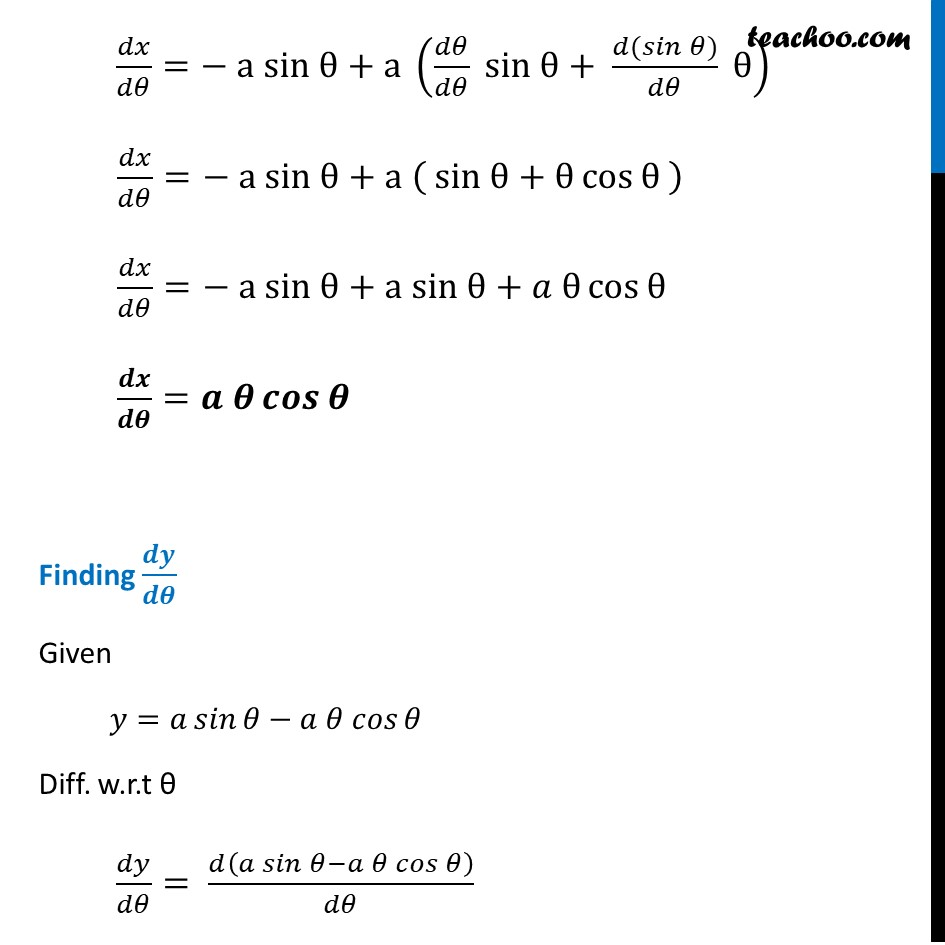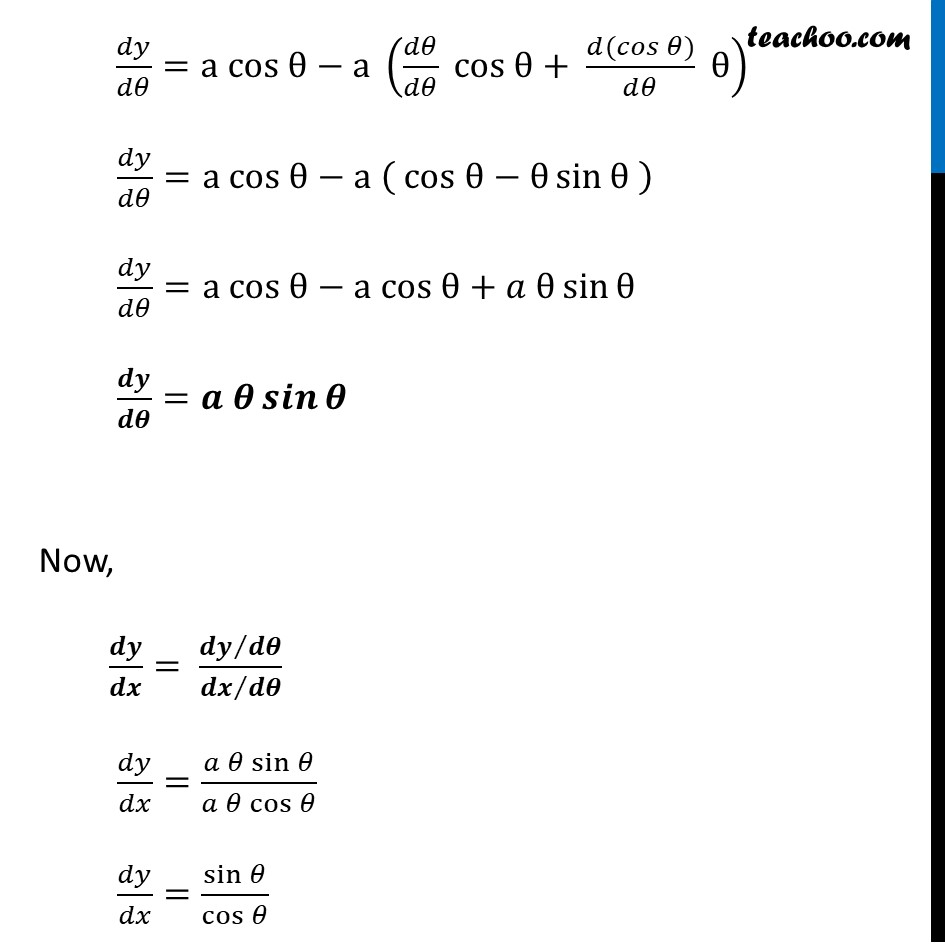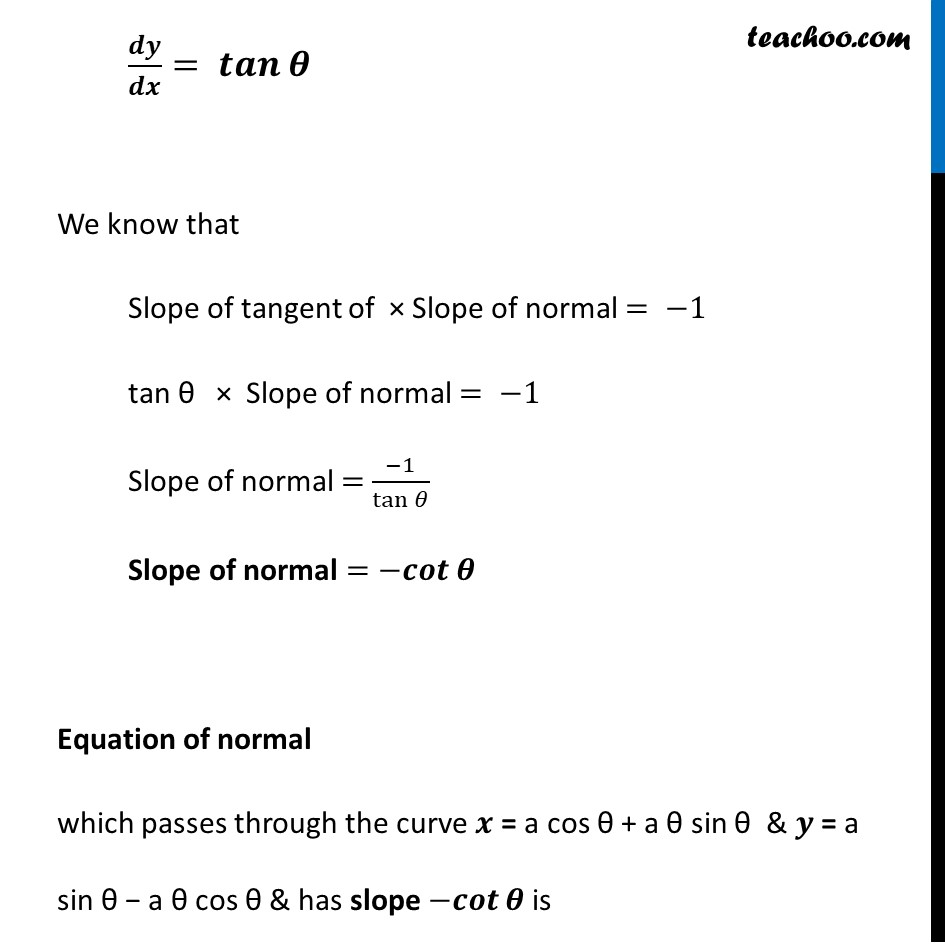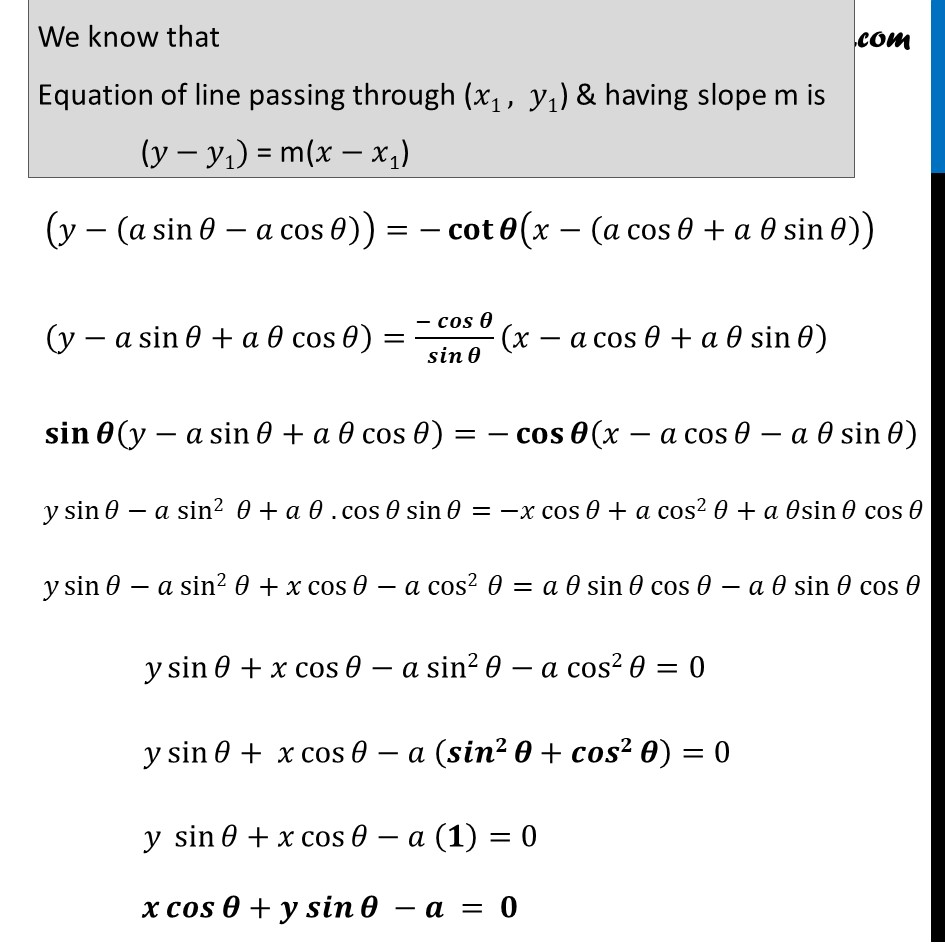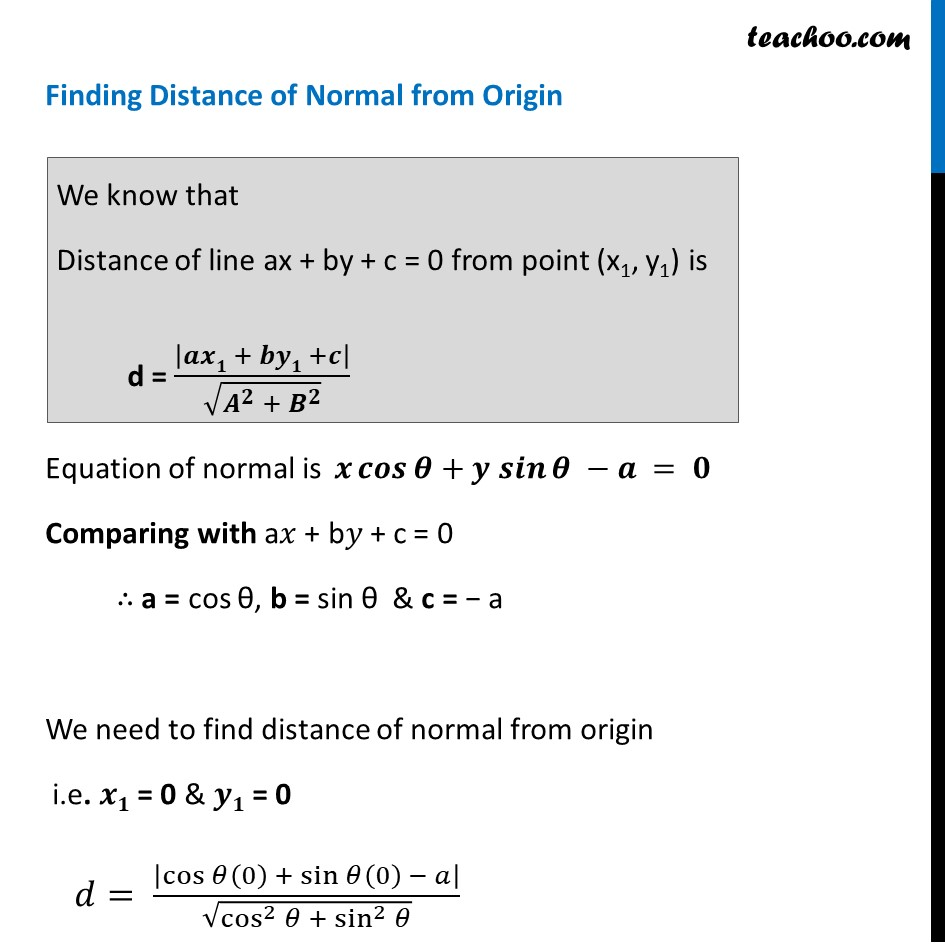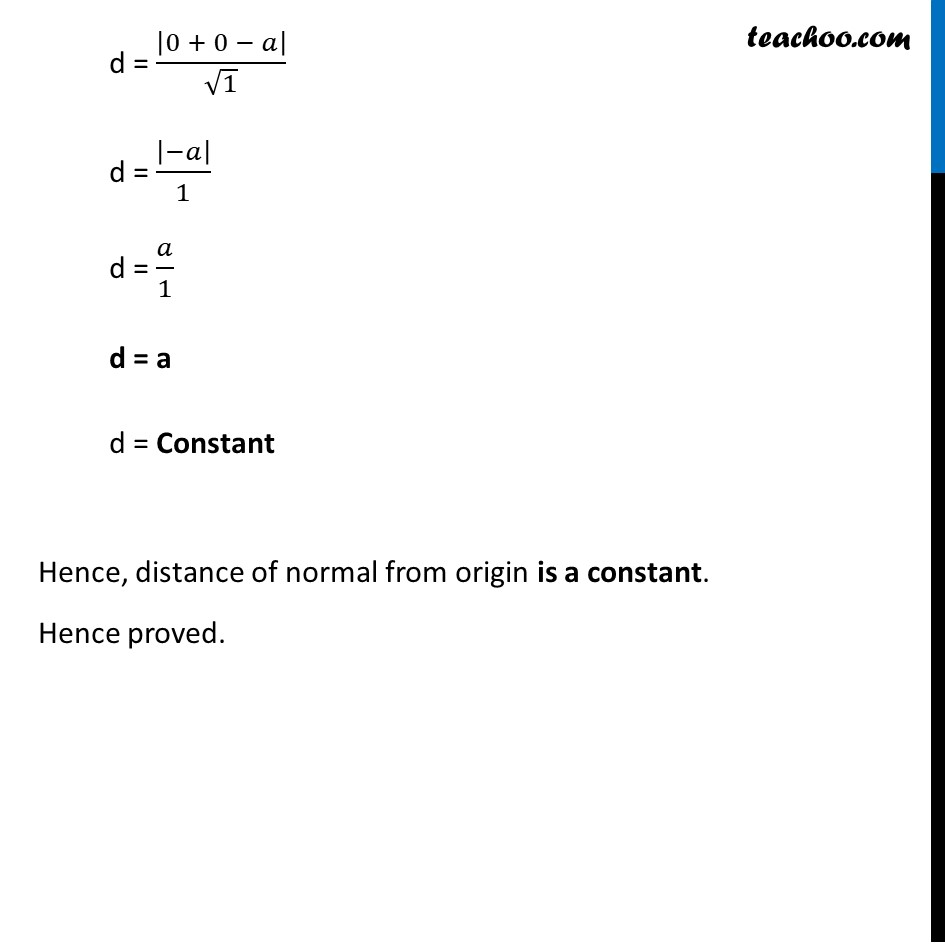Learn in your speed, with individual attention - Teachoo Maths 1-on-1 Class

### Transcript

Question 3 Show that the normal at any point θ to the curve x = a cos 𝜃 + a 𝜃 sin 𝜃, y = a sin 𝜃 – a 𝜃 cos 𝜃 is at a constant distance from the origin.Given curve 𝒙=𝑎 cos⁡𝜃+𝑎 𝜃 sin⁡𝜃 , 𝒚=𝑎 sin⁡𝜃– 𝑎 𝜃 cos⁡𝜃 We need to show distance of a normal from (0, 0) is constant First , calculating Equation of Normal We know that Slope of tangent is 𝑑𝑦/𝑑𝑥 𝒅𝒚/𝒅𝒙= (𝒅𝒚/𝒅𝜽)/(𝒅𝒙/𝒅𝜽) Finding 𝒅𝒙/𝒅𝜽 Given 𝑥=𝑎 cos⁡𝜃+𝑎 𝜃 sin⁡𝜃 Diff. w.r.t θ 𝑑𝑥/𝑑𝜃= 𝑑(𝑎 cos⁡𝜃 + 𝑎 𝜃 sin⁡𝜃 )/𝑑𝜃 𝑑𝑥/𝑑𝜃= (𝑑(𝑎 cos⁡𝜃))/𝑑𝜃 + (𝑑(𝑎𝜃 sin⁡𝜃))/𝑑𝜃 𝑑𝑥/𝑑𝜃 = 𝑎 (−sin⁡𝜃 )+𝑎 (𝑑(𝜃 sin⁡𝜃))/𝑑𝜃 Using product rule (u v)’ = u’ v + v’ u 𝑑𝑥/𝑑𝜃=− a sin θ+a (𝑑𝜃/𝑑𝜃 sin θ+ (𝑑(𝑠𝑖𝑛 𝜃))/𝑑𝜃 θ) 𝑑𝑥/𝑑𝜃=− a sin θ+a ( sin θ+θ cos⁡〖θ 〗 ) 𝑑𝑥/𝑑𝜃=− a sin θ+a sin θ+𝑎 θ cos⁡〖θ 〗 𝒅𝒙/𝒅𝜽=𝒂 𝜽 𝒄𝒐𝒔⁡〖𝜽 〗 Finding 𝒅𝒚/𝒅𝜽 Given 𝑦=𝑎 𝑠𝑖𝑛⁡𝜃−𝑎 𝜃 𝑐𝑜𝑠⁡𝜃 Diff. w.r.t θ 𝑑𝑦/𝑑𝜃= 𝑑(𝑎 𝑠𝑖𝑛⁡𝜃−𝑎 𝜃 𝑐𝑜𝑠⁡𝜃)/𝑑𝜃 𝑑𝑦/𝑑𝜃=a cos θ−a (𝑑𝜃/𝑑𝜃 cos θ+ (𝑑(𝑐𝑜𝑠 𝜃))/𝑑𝜃 θ) 𝑑𝑦/𝑑𝜃=a cos θ−a ( cos θ−θ sin⁡〖θ 〗 ) 𝑑𝑦/𝑑𝜃=a cos θ−a cos θ+𝑎 θ sin⁡〖θ 〗 𝒅𝒚/𝒅𝜽=𝒂 𝜽 𝒔𝒊𝒏⁡〖𝜽 〗 Now, 𝒅𝒚/𝒅𝒙= (𝒅𝒚∕𝒅𝜽)/(𝒅𝒙∕𝒅𝜽) 𝑑𝑦/𝑑𝑥=(𝑎 𝜃 sin⁡𝜃)/(𝑎 𝜃 cos⁡𝜃 ) 𝑑𝑦/𝑑𝑥=sin⁡𝜃/cos⁡𝜃 𝒅𝒚/𝒅𝒙= 𝒕𝒂𝒏⁡𝜽 We know that Slope of tangent of × Slope of normal = −1 tan θ × Slope of normal = −1 Slope of normal = (−1)/tan⁡𝜃 Slope of normal =−𝒄𝒐𝒕⁡𝜽 Equation of normal which passes through the curve 𝒙 = a cos θ + a θ sin θ & 𝒚 = a sin θ − a θ cos θ & has slope −𝒄𝒐𝒕⁡𝜽 is We know that Equation of line passing through (𝑥1 , 𝑦1) & having slope m is (𝑦−𝑦1) = m(𝑥−𝑥1) (𝑦−(𝑎 sin⁡𝜃−𝑎 cos⁡𝜃 ))=−𝐜𝐨𝐭⁡𝜽(𝑥−(𝑎 cos⁡𝜃+𝑎 𝜃 sin⁡𝜃 )) (𝑦−𝑎 sin⁡𝜃+𝑎 𝜃 cos⁡𝜃 )=(−𝒄𝒐𝒔⁡𝜽)/𝒔𝒊𝒏⁡𝜽 (𝑥−𝑎 cos⁡𝜃+𝑎 𝜃 sin⁡𝜃 ) 𝐬𝐢𝐧⁡𝜽(𝑦−𝑎 sin⁡𝜃+𝑎 𝜃 cos⁡𝜃 )=−𝐜𝐨𝐬⁡𝜽(𝑥−𝑎 cos⁡𝜃−𝑎 𝜃 sin⁡𝜃 ) 𝑦 sin⁡𝜃−𝑎 sin2 𝜃+𝑎 𝜃 .cos⁡𝜃 sin⁡𝜃=−𝑥 cos⁡𝜃+𝑎 cos2 𝜃+𝑎 𝜃sin⁡𝜃 cos⁡𝜃 𝑦 sin⁡𝜃−𝑎 sin2 𝜃+𝑥 cos⁡𝜃−𝑎 cos2 𝜃=𝑎 𝜃 sin⁡𝜃 cos⁡𝜃−𝑎 𝜃 sin⁡𝜃 cos⁡𝜃 𝑦 sin⁡𝜃+𝑥 cos⁡𝜃−𝑎 sin2 𝜃−𝑎 cos2 𝜃=0 𝑦 sin⁡𝜃+ 𝑥 cos⁡𝜃−𝑎 (𝒔𝒊𝒏𝟐 𝜽+𝒄𝒐𝒔𝟐 𝜽)=0 𝑦 sin⁡𝜃+𝑥 cos⁡𝜃−𝑎 (𝟏)=0 𝒙 𝒄𝒐𝒔⁡𝜽+𝒚 𝒔𝒊𝒏⁡𝜽 −𝒂 = 𝟎 We know that Distance of line ax + by + c = 0 from point (x1, y1) is d = |𝒂𝒙𝟏 + 𝒃𝒚𝟏 +𝒄|/√(𝑨^𝟐 + 𝑩^𝟐 ) Finding Distance of Normal from Origin Equation of normal is 𝒙 𝒄𝒐𝒔⁡𝜽+𝒚 𝒔𝒊𝒏⁡𝜽 −𝒂 = 𝟎 Comparing with a𝑥 + b𝑦 + c = 0 ∴ a = cos θ, b = sin θ & c = − a We need to find distance of normal from origin i.e. 𝒙𝟏 = 0 & 𝒚𝟏 = 0 𝑑= |cos⁡〖𝜃(0) + sin⁡〖𝜃(0) − 𝑎〗 〗 |/√(cos^2⁡𝜃 + sin^2⁡𝜃 ) d = |0 + 0 − 𝑎|/√1 d = |−𝑎|/1 d = 𝑎/1 d = a d = Constant Hence, distance of normal from origin is a constant. Hence proved.